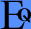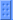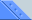More Skins Functions EMail BackGeneral BehaviorThis document explains exactly how Equalizer "understands" your expression. It can help you if you have any problems with it: First of all, Equalizer removes all spaces from your expression. "123+23/4" is the same as "1   2 3 +    23/ 4". Second, It replaces all curled brackets { } with normal bracket ( ). "(1+{3-{23+4))}" is the same as "(1+(3-(23+4)))". Note: square brackets [ ] are for absolute value. It replaces all "--" with a "+". Equalizer is smart enough to understand different functions of plus and minus. It distinguishes, for example, "3^-2" (3-2) from "3^2-2" (32-2). Same with all functions and operators. It understands unknown expressions as zero (I'm still working on that, don't worry). "Hello" will show the solution "0", "3/0" will also show zero. All trigonometry functions use radians. You can use the "deg" function to use angles of normal people. For absolute value use [ square brackets ]. Although you'd normally write it with pipes ( |abs value| ), it would be almost impossible for me to understand; so I decided to use [ ]. It may not function very well with extremely large or small numbers. It does understand short multiplying (ie: "3cos45"), but nothing with brackets (ie: "3(4+1)")Hot KeysAlt+C - Copy result to cliboard. Ctrl+Alt+C - Copy expression and result to cliboard ("1+2+3=6"). Alt+X - Clear the expression line. Ctrl+M - Minimize. Alt+F4 \ Ctrl+Q - Quit.